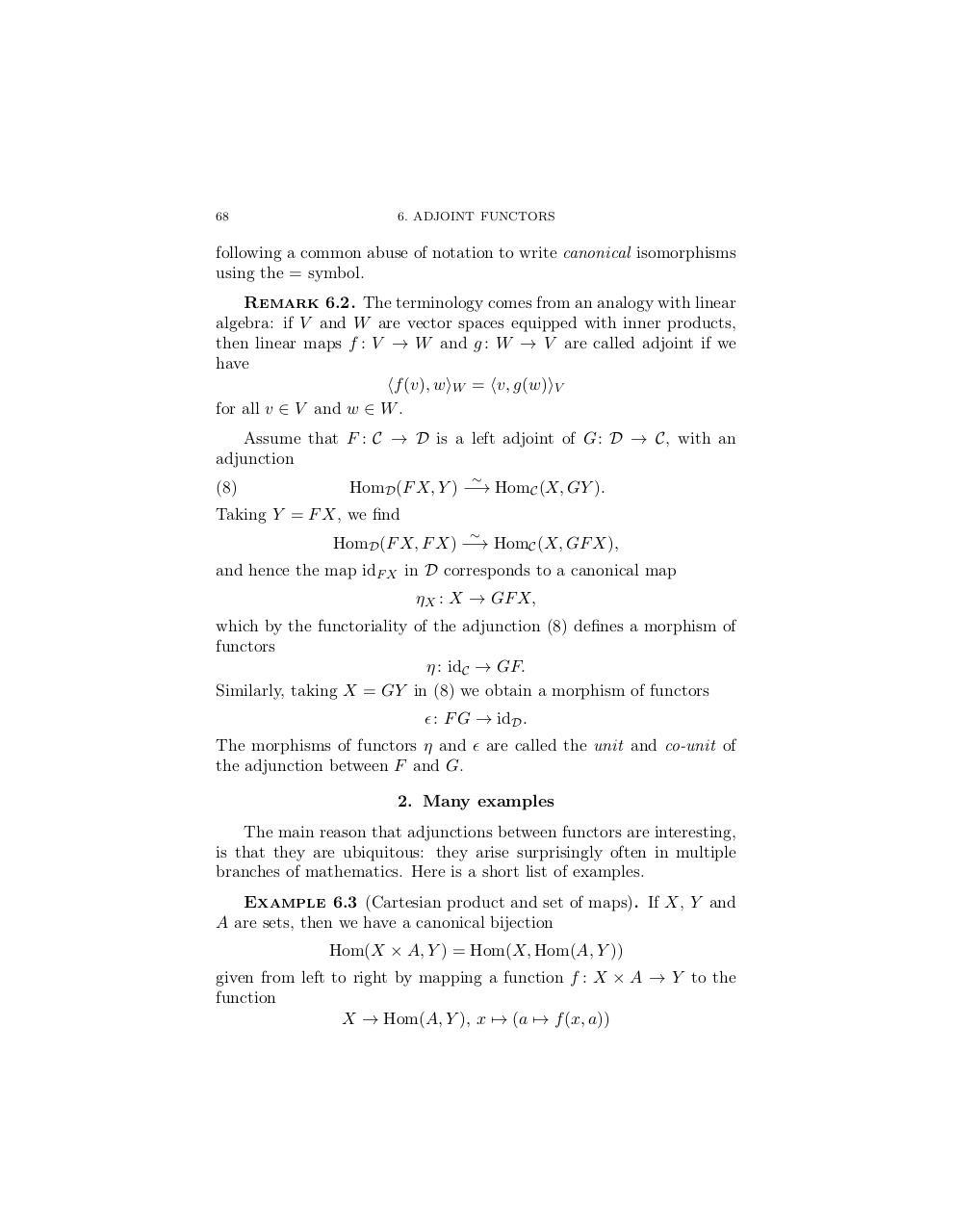# yoneda lenny.pdfPage 1 2 3 4 5 6 7 8 9

#### Text preview

68

following a common abuse of notation to write canonical isomorphisms
using the = symbol.
Remark 6.2. The terminology comes from an analogy with linear
algebra: if V and W are vector spaces equipped with inner products,
then linear maps f : V → W and g : W → V are called adjoint if we
have
hf (v), wiW = hv, g(w)iV
for all v ∈ V and w ∈ W .
Assume that F : C → D is a left adjoint of G : D → C, with an
(8)

HomD (F X, Y ) −→ HomC (X, GY ).

Taking Y = F X, we find

HomD (F X, F X) −→ HomC (X, GF X),
and hence the map idF X in D corresponds to a canonical map
ηX : X → GF X,
which by the functoriality of the adjunction (8) defines a morphism of
functors
η : idC → GF.
Similarly, taking X = GY in (8) we obtain a morphism of functors
 : F G → idD .
The morphisms of functors η and  are called the unit and co-unit of
the adjunction between F and G.
2. Many examples
The main reason that adjunctions between functors are interesting,
is that they are ubiquitous: they arise surprisingly often in multiple
branches of mathematics. Here is a short list of examples.
Example 6.3 (Cartesian product and set of maps). If X, Y and
A are sets, then we have a canonical bijection
Hom(X × A, Y ) = Hom(X, Hom(A, Y ))
given from left to right by mapping a function f : X × A → Y to the
function
X → Hom(A, Y ), x 7→ (a 7→ f (x, a))